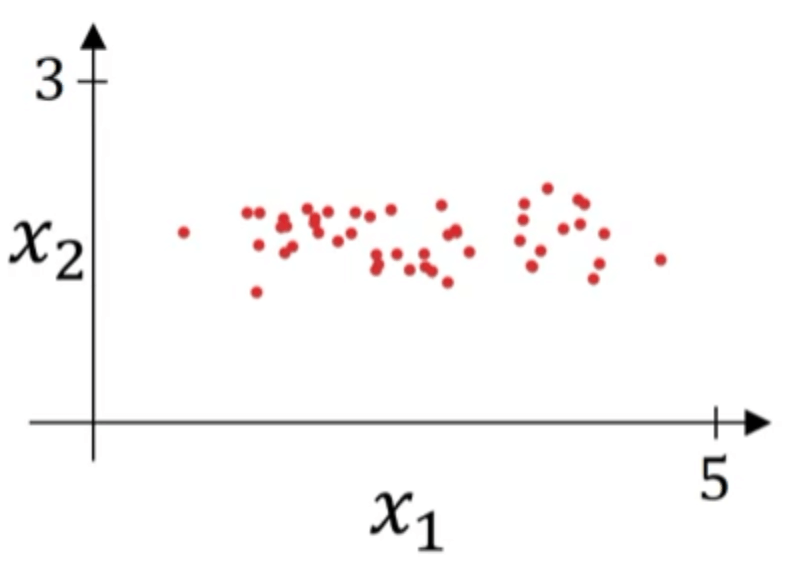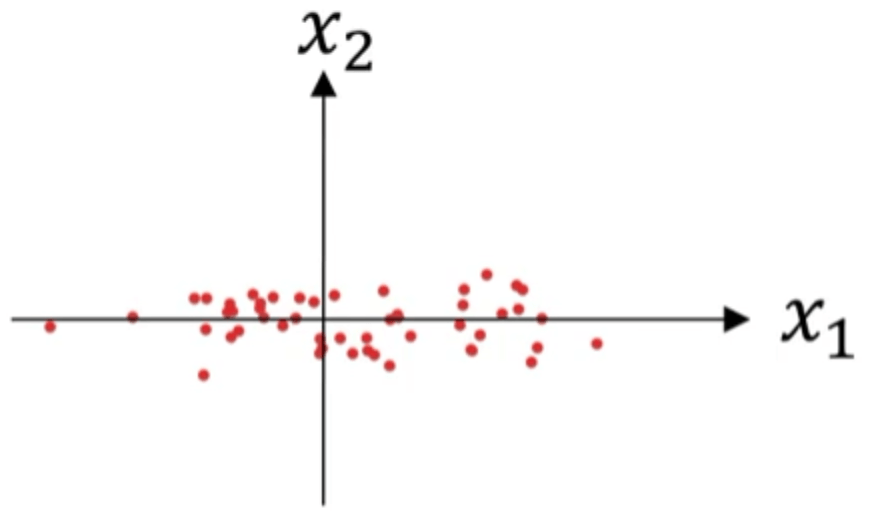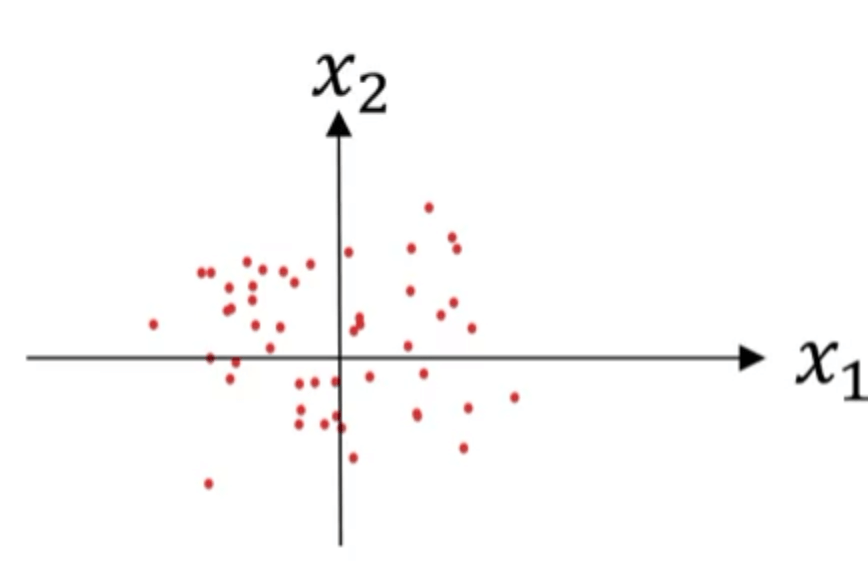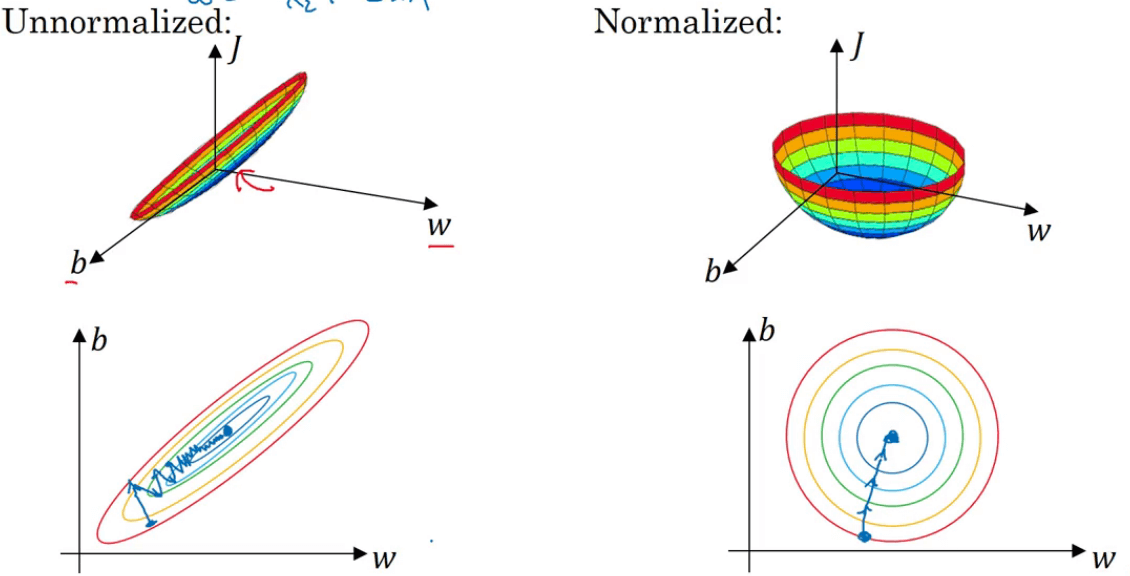# 【深度学习基础】第十二课：归一化输入

## 归一化输入

Posted by x-jeff on February 3, 2020

【深度学习基础】系列博客为学习Coursera上吴恩达深度学习课程所做的课程笔记。

# 2.归一化输入的方法## 2.1.零均值化

$\mu = \frac{1}{m} \sum_{i=1}^m x^{(i)}$ $x:=x-\mu$## 2.2.归一化方差

$\sigma^2 = \frac{1}{m} \sum_{i=1}^m [x^{(i)}]^2$ $x /= \sqrt{\sigma ^2+\epsilon}$⚠️记得用相同的$\mu$和$\sigma ^2$去归一化测试集，要和训练集保持一致。

# 3.归一化输入的原因👉如果特征值处于相似范围内（数量级差异并不大），那么归一化输入就显得不是那么重要了。